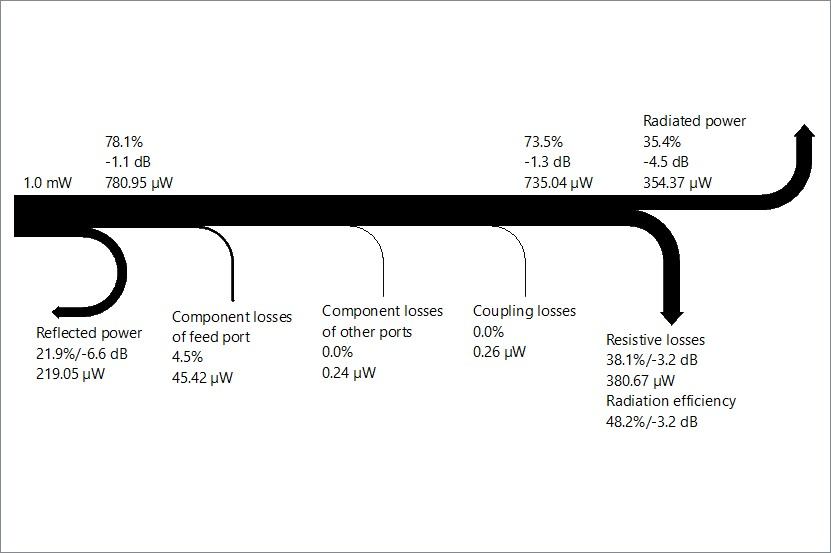# Optenni Lab: Power Balance Diagram

#### Unique tool providing a compact visualization of power loss contributors and total radiated power, including coupled power in a multiport system.The power balance diagram shows the various loss contributions in a multiantenna system.

Optenni Lab’s power balance diagrams are a unique way to understand the various loss mechanisms in the RF chain. The power balance diagram shows the reflected power, total component losses, coupling losses, resistive losses due to non-ideal radiation efficiency and finally the radiated power (for antenna applications) or the transmitted power to other ports (for non-antenna applications).

The losses can be shown relative to the input power in decibels or in percentage. If the input power is given, the loss contributions in terms of the absolute loss power are shown.

The power balance diagram is a handy tool to explain that the goal of the matching circuit design is not to minimize the reflected power but to maximize the radiated or transmitted power. If the optimization criteria would be the minimization of S11 (minimization of reflected power), the optimizer might generate matching solutions where the component losses would consume most of the power. In contrast, when the total efficiency is optimized, Optenni Lab tries to balance the different loss mechanisms so that maximal radiated power is obtained.